# How to VLOOKUP with numbers and text in Excel

In this article, we will learn about how to use VLOOKUP function with numbers and text in Excel.

VLOOKUP just searches for the row of a given value in the first column of a table and returns asked column’s value in that row.

Syntax:

=VLOOKUP(lookup_value, table_array, col_index_number, [range_lookup])

Lookup_value: The value by which you want to search in the first column of Table Array.
Table_array: The Table in which you want to look up/search
col_index_number: The column number in Table Array from which you want to fetch results.
[range_lookup]: FALSE if you want to search for the exact value, TRUE if you want an approximate match.

Let’s understand this function using it an example.

Here we have a Source Table and Vlookup Information and we need to find the details of a person using Define names option in Excel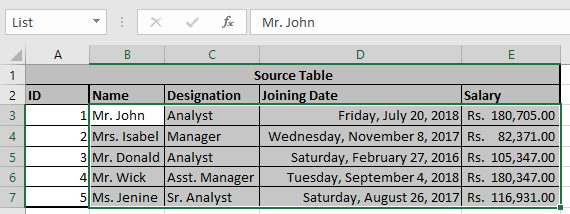Here we defined the name “List” to the selected table above using define name option.

And name “Table” using Define name as shown below.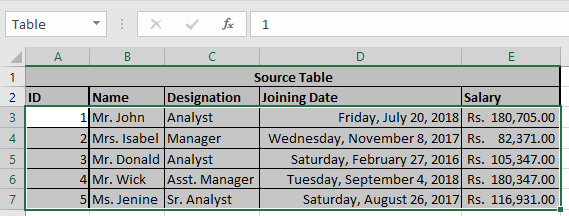Now we need to find some values from the table using VLOOKUP formula.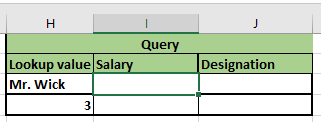We have assigned names to the lookup values. Mr. wick as “Name”.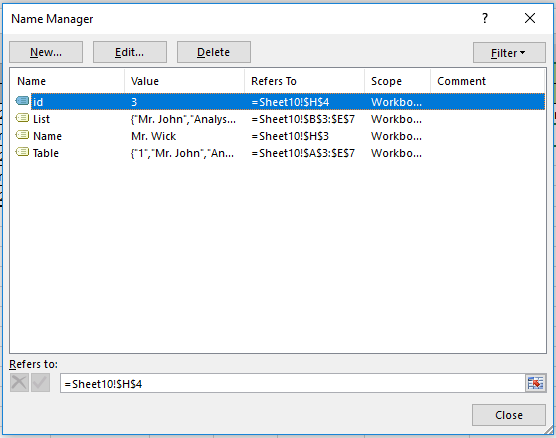To find value for Mr. Wick, use the Vlookup formula

=VLOOKUP(Name,List,4,0)
=VLOOKUP(Name,List,2,0)

Explanation:
The Formula looks up for the Name which is Mr. Wick in the table named List and returns its corresponding results from the table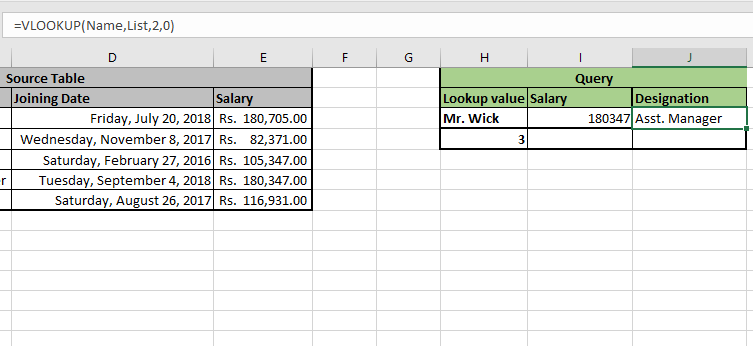As you can see here, the formula returns the results for Mr. Wick.

Now we need to find values for “id” which is 3 from the table using vlookup formula.We have assigned names to the lookup values.3 as “id”.

To find the value for ID 3, use the Vlookup formula

=VLOOKUP(id,Table,5,0)
=VLOOKUP(id,Table,3,0)

Explanation:
The Formula looks up for the id which is 3 in the Table and returns its corresponding results from the Table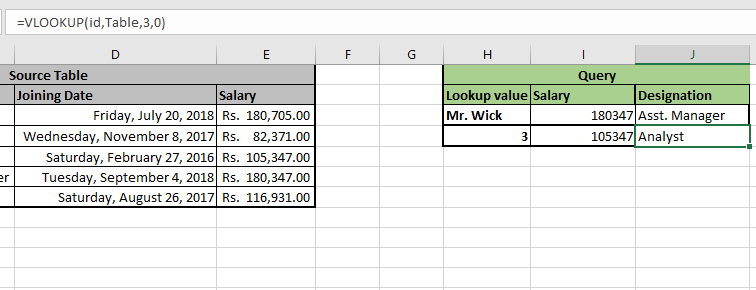As you can see here, the formula returns the results for Mr. Wick.

Hope you understood how to use VLOOKUP formula with numbers and Text in Excel. Explore more articles on Excel VLOOKUP function on Exceltip.com here. Please feel free to share your queries with us in the comment box below. We will help you.

Related Articles:

How to use the INDEX Function

How to use the VLOOKUP Function in Excel

How to use the SMALL function in Excel

How to use the IF Function in Excel

How to Lookup Value with Multiple Criteria in Excel

Vlookup Top 5 Values with Duplicate Values Using INDEX-MATCH in Excel

How to VLOOKUP with numbers and text in Excel

Popular Articles:

50 Excel Shortcuts to Increase Your Productivity

How to use the VLOOKUP Function in Excel

How to use the COUNTIF function in Excel

How to use the SUMIF Function in Excel

Terms and Conditions of use

The applications/code on this site are distributed as is and without warranties or liability. In no event shall the owner of the copyrights, or the authors of the applications/code be liable for any loss of profit, any problems or any damage resulting from the use or evaluation of the applications/code.